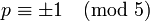# Linear representation theory of alternating group:A6

## Contents

View linear representation theory of particular groups | View other specific information about alternating group:A6

## Summary

Item Value
Degrees of irreducible representations over a splitting field (such as$\mathbb{C}$ or$\overline{\mathbb{Q}}$) 1,5,5,8,8,9,10
grouped form: 1 (1 time), 5 (2 times), 8 (2 times), 9 (1 time), 10 (1 time)
maximum: 10, lcm: 360, number: 7, sum of squares: 360
Ring generated by character values$\mathbb{Z}[2\cos(2\pi/5)] = \mathbb{Z}[(1 + \sqrt{5})/2]$
Minimal splitting field, i.e., field of realization of all irreducible representations (characteristic zero)$\mathbb{Q}(\sqrt{5})$
Quadratic extension of$\mathbb{Q}$
Same as field generated by character values
Orbits of irreducible representations under action of automorphism group orbits of size 1 for representations of degree 1,9,10; orbits of size two for degree 5 and degree 8 representations (the degree 8 representations are interchanged under conjugation by an odd permutation; the degree 5 representations are interchaged by an automorphism that is outer for$S_6$ as well)
Orbits of irreducible representations under action of Galois group orbits of size 1 for representations of degree 1,5,5,9,10; orbit of size two for degree 8 representations (automorphism$\sqrt{5} \mapsto -\sqrt{5}$)
Minimal splitting field in prime characteristic$p \ne 2,3,5$ Case$p \equiv \pm 1 \pmod 5$: prime field$\mathbb{F}_p$
Case$p \equiv \pm 2 \pmod 5$: quadratic extension$\mathbb{F}_{p^2}$ of$\mathbb{F}_p$
Smallest size splitting field field:F11
Degrees of irreducible representations over the rational numbers 1,5,5,9,10,16

## Family contexts

Family name Parameter values General discussion of linear representation theory of family
alternating group 6 linear representation theory of alternating groups
projective special linear group of degree two over a finite field of size$q$$q = 9$, i.e., field:F9, i.e., field of nine elements, so the group is$PSL(2,9)$ linear representation theory of projective special linear group of degree two over a finite field

## GAP implementation

The degrees of irreducible representations can be found using GAP's CharacterDegrees and AlternatingGroup functions:

gap> CharacterDegrees(AlternatingGroup(6));
[ [ 1, 1 ], [ 5, 2 ], [ 8, 2 ], [ 9, 1 ], [ 10, 1 ] ]

This means that there is 1 degree 1 irreducible, 2 degree 5 irreducibles, 2 degree 8 irreducibles, 1 degree 9 irreducible, and 1 degree 10 irreducible representation.

The characters of irreducible representations can be computed using GAP's CharacterTable function:

gap> Irr(CharacterTable(AlternatingGroup(6)));
[ Character( CharacterTable( Alt( [ 1 .. 6 ] ) ), [ 1, 1, 1, 1, 1, 1, 1 ] ),
Character( CharacterTable( Alt( [ 1 .. 6 ] ) ), [ 5, 1, 2, -1, -1, 0, 0 ] ),
Character( CharacterTable( Alt( [ 1 .. 6 ] ) ), [ 5, 1, -1, 2, -1, 0, 0 ] ),
Character( CharacterTable( Alt( [ 1 .. 6 ] ) ), [ 8, 0, -1, -1, 0, -E(5)-E(5)^4,
-E(5)^2-E(5)^3 ] ), Character( CharacterTable( Alt( [ 1 .. 6 ] ) ),
[ 8, 0, -1, -1, 0, -E(5)^2-E(5)^3, -E(5)-E(5)^4 ] ),
Character( CharacterTable( Alt( [ 1 .. 6 ] ) ), [ 9, 1, 0, 0, 1, -1, -1 ] ),
Character( CharacterTable( Alt( [ 1 .. 6 ] ) ), [ 10, -2, 1, 1, 0, 0, 0 ] ) ]